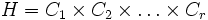# Abelian direct factor implies potentially verbal in finite

This article gives the statement and possibly, proof, of an implication relation between two subgroup properties, when the big group is a finite group. That is, it states that in a Finite group (?), every subgroup satisfying the first subgroup property (i.e., Abelian direct factor (?)) must also satisfy the second subgroup property (i.e., Potentially verbal subgroup (?)). In other words, every Abelian direct factor of finite group is a potentially verbal subgroup of finite group.
View all subgroup property implications in finite groups$|$ View all subgroup property non-implications in finite groups$|$ View all subgroup property implications$|$ View all subgroup property non-implications

## Statement

Suppose$G$ is a finite group and$H$ is a direct factor of$G$ such that$H$ is abelian as a group. Then, there exists a group$K$ containing$G$ such that$H$ is a verbal subgroup.

## Facts used

1. structure theorem for finitely generated abelian groups

## Proof

Given: A finite group$G$ with direct factor$H$. Let$L$ be a normal complement to$H$ in$G$, so$G \cong H \times L$.

To prove: There exists a group$K$ containing$G$ such that$H$ is a verbal subgroup of$K$.

Proof: If$H$ is trivial, we can set$K = G$ and be done. So, we can assume$H$ is nontrivial.

1. By fact (1), we can write$H$ as a direct product of cyclic groups, say$H = C_1 \times C_2 \times \dots \times C_r$, where each$C_i$ is cyclic of prime power order, say$p_i^{k_i}$.
2. For each prime$p$, let$c(p)$ be a positive integer greater than the largest power of$p$ dividing the order of$L$. (Note that the same$p$ may repeat among multiple$p_i$s).
3. Now consider the group$M = D_1 \times D_2 \times \dots \times D_r$ where each$D_i$ is cyclic of order$p_i^{k_i + c(p_i)}$. Treat$H$ as the subgroup of$M$ obtained by embedding each$C_i$ naturally into the corresponding$D_i$.
4. Define$K = M \times L$, with$G$ embedded in$K$ using the embedding of$H$ in$M$ provided above.
5. Let$n = \prod p^{c(p)}$ where the product is over the primes$p$ dividing the order of$H$. Then, it is clear that the set of$n^{th}$ powers in$K$ is precisely$H$. In particular,$H$ is a verbal subgroup of$K$.